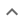•# Factoring multivariable polynomials

We think you wrote:

###This solution deals with factoring multivariable polynomials.

Solution found(2x+3y)*(2x+5y)

## Step by Step Solution## Step  1  :

#### Equation at the end of step  1  :

  ((4 • (x2)) +  16xy) +  (3•5y2)
Step  2  :

#### Equation at the end of step  2  :

  (22x2 +  16xy) +  (3•5y2)


## Step  3  :

#### Trying to factor a multi variable polynomial :

Factoring    4x2 + 16xy + 15y2

Try to factor this multi-variable trinomial using trial and error

Found a factorization  :  (2x + 3y)•(2x + 5y)

## Final result :

  (2x + 3y) • (2x + 5y)